Home > INT3 > Chapter 7 > Lesson 7.1.4 > Problem7-44

7-44.
1.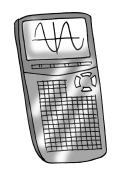Using your calculator, solve the equations below. Round your answers to the nearest 0.001. Homework Help ✎

1. x6 = 125

2. x3.8 = 240

3. x–4 = 100

4. (x + 2)3 = 65

5. 4(x – 2)12.5 = 2486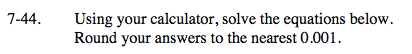Undo powers with fractional exponents. If x is not in the exponent, you should not use logs.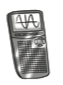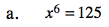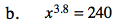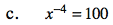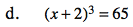x = 2.021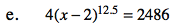Divide by 4 first.

x = 3.673